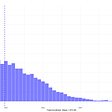# Google Colab and Auto-sklearn with Profiling

`X, y, coeff = make_regression(    n_samples=1000,    n_features=100,    n_informative=5,    noise=0,    shuffle=False,    coef=True)`
`import autosklearn.regressionautoml = autosklearn.regression.AutoSklearnRegressor(    time_left_for_this_task=300,    n_jobs=-1)automl.fit(    X_train_transformed,    df_train["label"])`
`predictions = automl.predict(X_train_transformed)r2_score(df_train["label"], predictions)>> 0.999predictions = automl.predict(X_test_transformed)r2_score(df_test["label"], predictions)>> 0.999`

--

--

--

Love podcasts or audiobooks? Learn on the go with our new app.

## Exploring natural disasters with Earth Map: Sierra Bermeja, a ‘sixth generation wildfire’ in Spain## 5 Reasons Why Numbers Are Misleading## Comparison Between Python and R Visualizations## Entropy; A method for Data Science & Machine Learning## Significance testing for model estimates: Wald test## Using TiNN the Tiny Neural Network in C With Python Ctypes## Why Finding ‘Why’ Is Difficult## How to use Cosine Similarity and the Tanimoto Coefficient## Pharmaceutical Sales Prediction using a deep learning model